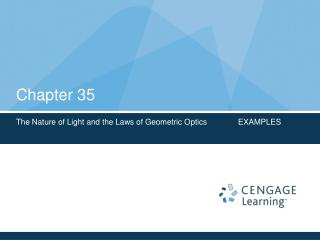DownloadDownload PresentationChapter 35

# Chapter 35

Télécharger la présentation## Chapter 35

- - - - - - - - - - - - - - - - - - - - - - - - - - - E N D - - - - - - - - - - - - - - - - - - - - - - - - - - -
##### Presentation Transcript

1. Chapter 35 The Nature of Light and the Laws of Geometric Optics EXAMPLES

2. Example 35.1 Double Reflection • Two mirrors make an angle of 120o with each other. An incident ray strikes the mirror M1 at an angle of 65o to the normal. Find the direction of the ray after it is reflected from mirror M2. • The reflected ray is directed toward the mirror M2. Making an angle of 90o 65o = 25o with the horizontal. • From the triangle made by the first reflection and the two mirrors: 180o 120o 25o = 35o (angle of the first reflected ray with M2. • Therefore, this ray makes an angle of 55o with the normal to M2. • From the law of reflection the second reflected ray makes an angle of 55o with the normal to M2.

3. Example 35.2 Double Reflection (2nd part) • From Example 35.1 if the rays are extended behind the mirrors, they cross at 60o, so that the overall change in direction of the light ray is 120o (same angle between mirrors). If the angle between mirrors is changed, is the overall change in the direction of the light ray always equal to the angle between the mirrors? • NO! By using the law of reflection and the sum of the interior angles of a triangle:  = 180o (90o )   = 90o+    . • From the highlighted triangle:  + 2 + 2 (90o ) =180o  = 2(  )

4. Example 35.2 Double Reflection, final • The change in direction of the light ray is the angle  = 180o    = 180o 2(  )   = 180o 2[  (90o+   )]   = 360o 2 • If  = 120o  = 120o • If  = 90o  = 180o (RETROREFLECTION) • Applications:

5. Quick Quiz 35.2 Following the Reflected and Refracted Rays • Ray  is the incident ray • Ray  is the reflected ray • Ray  is refracted into the Lucite block • Ray  is internally reflected in the block • Ray  is refracted as it enters the air from the block

6. Example 35.3 Angle of Refraction for Glass • Light of a wavelength 589 nm is refracted into a crown glass slab with1 = 30.0o. (A). Find the angle of refraction 2 = ? • n1 = 1.00 and n2 = 1.52 (from Table 35.1) • From eqn. (35.8) Then: 2 = sin-1(n1 / n2) sin 1 = 19.2o • The ray bends toward the normal, as expected

7. Example 35.3 Angle of Refraction for Glass, 2 (B). Find the speed of the light once enters the glass. From eqn. (35.4) (C). What is the wavelength of this light in the glass? From eqn. (35.7)

8. Example 35.4 Measuring n from a Prism • The minimum angle of deviation(δmin) for a prism occurs when the incident angle 1is such that the refracted ray inside the prism makes the same angle with the normal to the two prism faces. • Obtain an expression for the index of refraction of the prism material.

9. Example 35.4 Measuring n from a Prism, cont. • From the geometry: • From Snell’s Law (with n = 1 for air):

10. Example 35.5 Find Critical Angle C • Find C for an Air-Water boundary. n1 = 1.33 and n2 = 1.00

11. Example 35.6 A view from the Fish’s Eye • A fish in a still pond looks upward toward the water’s surface. What does it see? • Using the result from Example 35.5 • At an angle less than C (48.8°) the fish can see out of the water • At 48.8°the light has to skim along the water’s surface before been refracted, so the fish can see the whole shore of the pond. • At an angle greater than C (48.8°) the fish sees a reflection of the bottom of the pond.

12. Example 35.7 Light Passing Through a Slab • (A). A light beam passes from medium 1 to medium 2 with the latter medium being a thick slab of material whose index of refraction is. • Show that the beam emerging into medium 1 from the other side is parallel to the incident beam. • Apply Snell’s law to the upper surface: • Apply Snell’s law to the lower surface: • Therefore:

13. Example 35.7 Light Passing Through a Slab, 2 • (B). If the thickness t of the slab is doubled, what does happen to the offset distance d? • Find an expression for a from the yellow triangle: • Find an expression for d from the red triangle: • Combine these equations to find an expression for d in function of t: • Conclusion between d and t: • The angles are only related to index of refraction • (constants), so . • Therefore: If t doubles, so does d !

14. Material for the Midterm • Material from the book to Study!!! • Objective Questions: 8-12 • Conceptual Questions: 6-8 • Problems: 6-8-15-16-20-37-39